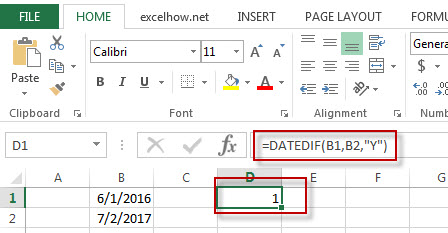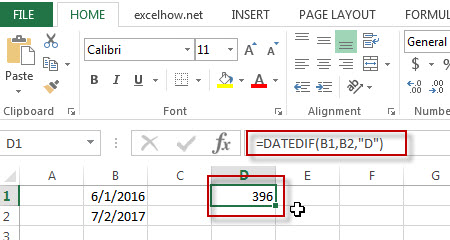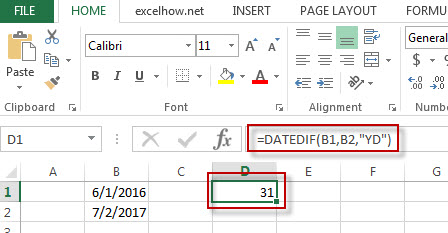# Excel DatedIF Function

This post will guide you how to use Excel DATEDIF function with syntax and examples in Microsoft excel.

### Description

The Excel DATEDIF function returns the number of days, months, or years between tow dates.

The DATEDIF function is a build-in function in Microsoft Excel and it is categorized as a DATE and TIME Function.

The DATEDIF function is available in Excel 2016, Excel 2013, Excel 2010, Excel 2007, Excel 2003, Excel XP, Excel 2000, Excel 2011 for Mac.

### Syntax

The syntax of the DATEDIF function is as below:

=DATEDIF (start_date,end_date,unit)

Where the DATEDIF function arguments are:
Unit –  the type of information that you want to return.
Note: The index_num value must be a number between 1 and 29.

• “Y”– The number of complete years
• “M”– The number of complete months
• “D”– The number of days
• “MD”– The difference between the days in start_date and end_date.
• “YM”– The difference between the months in start_date and end_date
• “YD”– The difference between the days(the years of dates are ignored)

### Example

The below examples will show you how to use Excel DATEDIF Function to return the difference between two dates.

#1 =DATEDIF(B1,B2,”Y”)Note: the above excel formula will return the number of complete year as “1”between start_date(6/1/2016) and end_date(07/2/2017).

#2 =DATEDIF(B1,B2,”D”)Note: the above formula will return the number of days(396) between 6/1/2016 and 7/2/2017.

#3 =DATEDIF(B1,B2,”YD”)Note: the above formula will return 31 days between 6/1 and 7/2, ignoring the years of dates.